# `1. What it does?`

Computes Temperature Compensated Viscosity, which is assumed to have an exponential trend.

# `2. Application`

Bromine, Acetone, Bromoform, Pentane, Bromobenzene

# `3. Math`

Below is find the mathematical formula for the viscosity exponential model.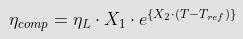The SRV and SMET are together a powerful tool in the measurement of inline viscosity and temperature.

Requirements:

• The viscosity of the fluid at different temperatures.
• Input the coefficients X1, X2, and Tref (Reference Temperature).
`3.1. How SRV measurements will be different from laboratoryinstruments for non-newtonian fluids due to shear rate?`

Many, if not most, fluids exhibit non-Newtonian behavior. A fluid is said to be non-Newtonian when its measured viscosity depends on the shear rate with which the measurement is conducted.

Viscosity is defined as the ratio of shear stress to shear rate when a fluid is subjected to uniform shearing. Figure 1 displays this behavior.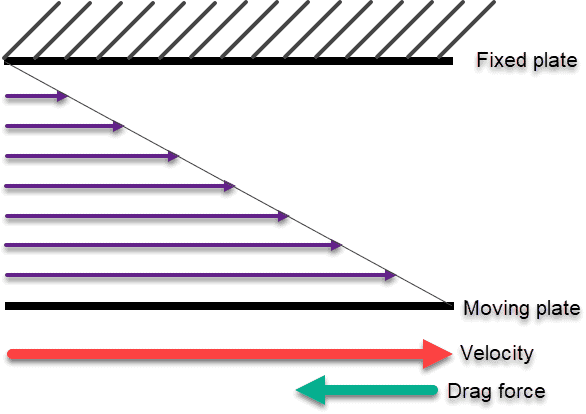Figure 1. A Newtonian fluid is sheared between two parallel plates.

Now that we have defined what is viscosity, we can compare the behavior of both Newtonian and Non-Newtonian

 Newtonian Fluid Non-Newtonian Fluid Changing the force, you apply to the fluid will not change its viscosity. Have viscosities that change according to the amount of force that is applied to the fluid. The viscosity remains constant as the force applied changes The viscosity changes as the force applied changes. The relation between shear stress and the shear rate is linear, passing through the origin, the constant of proportionality being the coefficient of viscosity. The relation between the shear stress and the shear rate is different and can even be time-dependent (Time-Dependent Viscosity) – a constant coefficient of viscosity cannot be defined

Table 1. Comparison table between Newtonian vs non-Newtonian fluids.

One kind of non-Newtonian behavior that is common to many fluids is that the measured viscosity decreases as the shear rate of the viscometer go up. This is called shear thinning behavior.

For our initial question, we can then understand that most fluids exhibit a non-linear relationship between shear stress and shear rate. That means that the measured viscosity is dependent on the type of viscometer used for the measurement. The SRV has a much higher shear rate than most rotational, capillary, and efflux cup viscometers. Therefore, the SRV will often show a substantially different viscosity than will a laboratory rotational instrument.

# `4. Checking the data`

The exponential model for viscosity needs coefficients X1 and X2 of the exponential model, those coefficients are calculated with the viscosity data of the fluid at different temperatures.

The following steps outline the correct procedure for data analysis:

`4.1. Known viscosity at reference temperature: This is obtained by taking measurements of viscosity at different temperatures including the reference temperature that is needed. Reference temperature and compensated viscosity desired are highlighted in blue.`
 Temp [C] Viscosity [Cp] 75 32.8 70 46.5 65 54 60 67.5 55 80.25 50 98.55 45 123.5 15 519.75

Table 2. Data points from inline measurements.

`4.2. Plot data points Viscosity vs Temperature. to study the behavior of the data. In this scenario, the data behaves as an exponential curve.`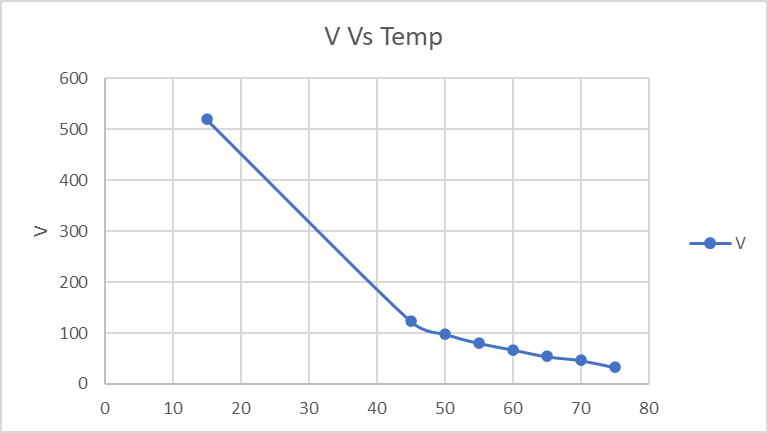Figure 2. Plot chart Viscosity vs Temperature.

`4.3. Calculate the relationship between compensated viscoisty and measured viscosity (Table 1)`
 V'= (Comp. Visco/Visco) @30° Results V'= (519.75/32.8) 15.846 V'= (519.75/46.5) 11.177 V'= (519.75/54) 9.625 V'= (519.75/67.5) 7.700 V'= (519.75/80.25) 6.477 V'= (519.75/98.55) 5.274 V'= (519.75/123.5) 4.209 V'= (519.75/519.75) 1.000

Table 3. Compensated Viscosity calculation table.

`4.4. Calculate the difference between Temperature reference and measured temperature(Table 1).`
 T' = T-Tref Results T' =75-15 60 T' =70-15 55 T' =65-15 50 T' =60-15 45 T' =55-15 40 T' =50-15 35 T' =45-15 30 T' =15-15 0

Table 5. Variation to the reference temperature.

`4.5. Coefficients estimation`

Use an exponential trendline to find X1 and X2 values or make a regression analysis to find the coefficients.

• Plot the V’ (Comp. Visco/Visco @30°) vs T' (T-Tref)
 V'= (Comp. Visco/Visco) @30° T' = T-Tref 15.846 60 11.177 55 9.625 50 7.700 45 6.477 40 5.274 35 4.209 30 1.000 0

Table 6. Datapoints for Viscosity and temperature referenced to known values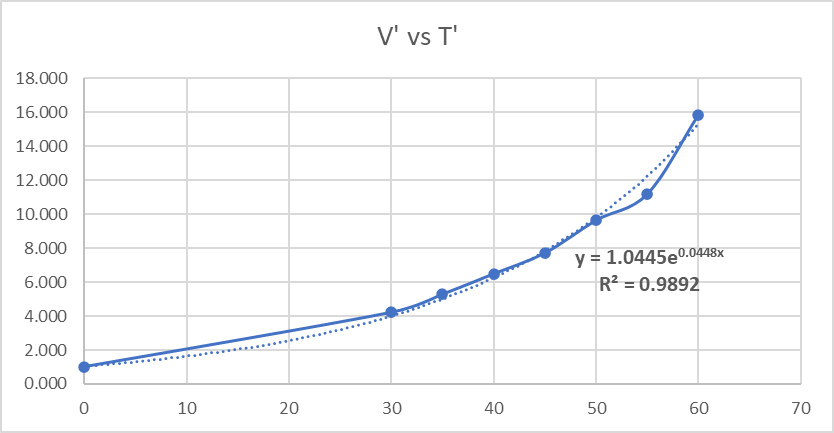Figure 3. Plot V´ vs T´ to find the correct coefficients to be used with the mathematical model

From this plot, we can determine the formula and R2 parameter, both are good results for the implementation of the Mathematical model.

With the exponential formula with form Y= X1*e(X2*T), the coefficients X1 and X2 result in X1=1.0445 and X2=0.0448.

All the input parameters are ready for the next step, the SMET will allow the temperature compensated viscosity.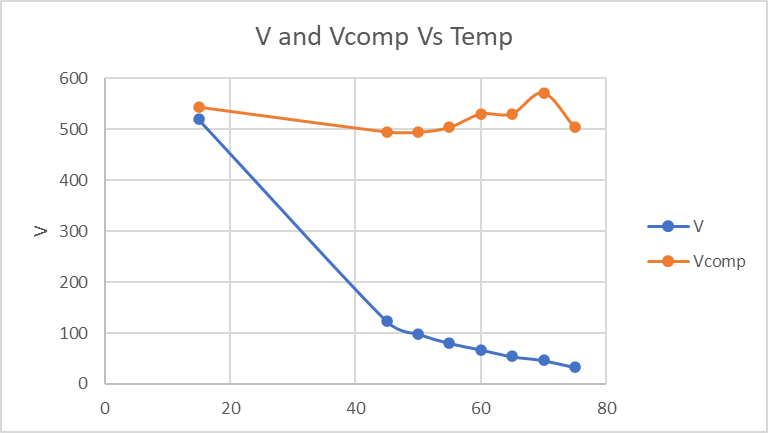Figure 4. Expected results when applying the mathematical model.

# `5. How to load models into the SMET?`

The calculation tab allows configuring the SMET to run mathematical models for Viscosity, Density, and Concentration. Expert mode is required to be enabled for this.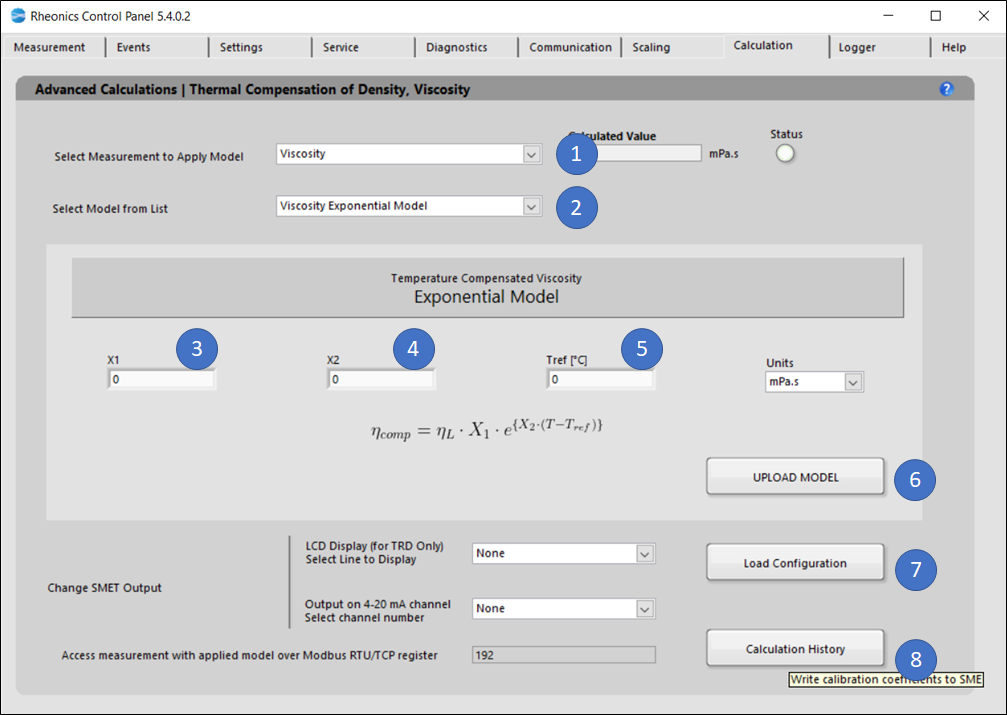Figure 5. Calculation tab for the exponential model of viscosity.

The following steps are to load the models into SMET:

1. In the Dropdown list “Select Measurement to Apply Model” select. That will modify the “Select Model from List” dropdown menu values. Select Measurement application (Viscosity)
2. In “Select Model from list” select the model you would like to use for the specific parameter. When you select the model, you will get more information about the specific equation used and the coefficients you will need to apply to that model.  Select “Viscosity Exponential model”
3. Input X1 coefficient calculated in Section 5.
4. Input X2 coefficient calculated in Section 5.
5. Input Reference temperature used to find the coefficients models.
6. Click “Upload Model” to load the model settings into SMET. The button should turn GREEN for a couple of seconds if the action was successful or turn RED if the operation was Rheonics Control Panel Software unsuccessful. This action will refresh the values for the Uncompensated and Compensated indicators. Here you can verify if the model is calculating the expected values. If not, you can correct and repeat the process.
7. Click “Load Configuration” to update the display and channel configurations in SMET.
`5.1. LCD Display`

Select the line to display the parameter: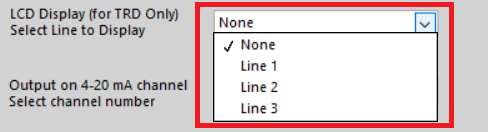Figure 6. Selecting read line for LCD display.

Changes can be verified on the “Communication” Tab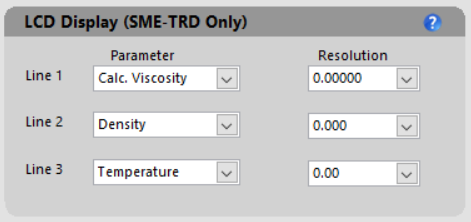Figure 7. The communication tab has loaded concentration parameters.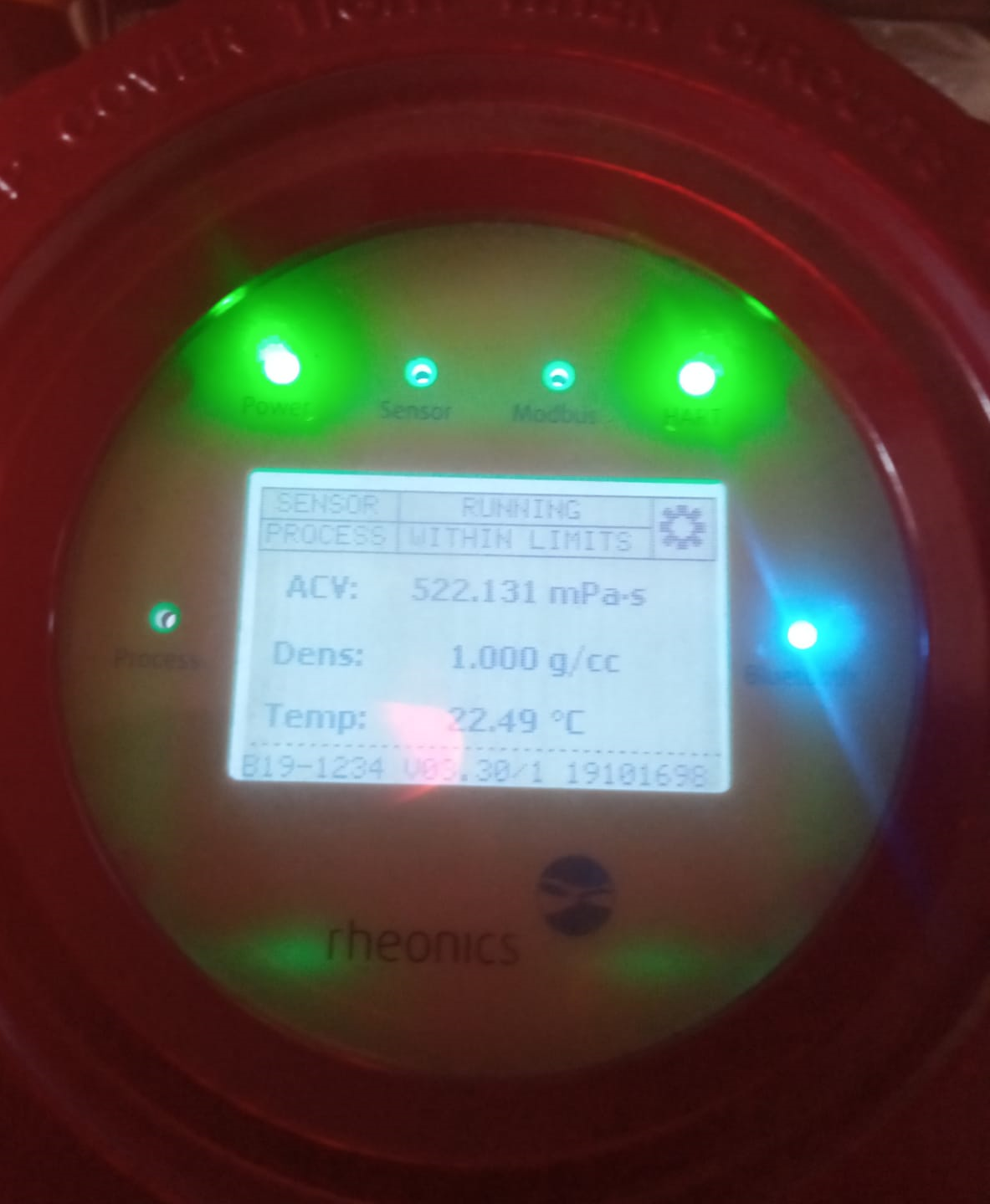Figure 8. Parameters loaded into the SMET-TRD.

`5.2. Analog outputs`

Select analog channel to send data, this can be verified in the “Service” Tab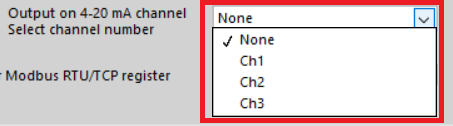Figure 9. Selecting analog output channel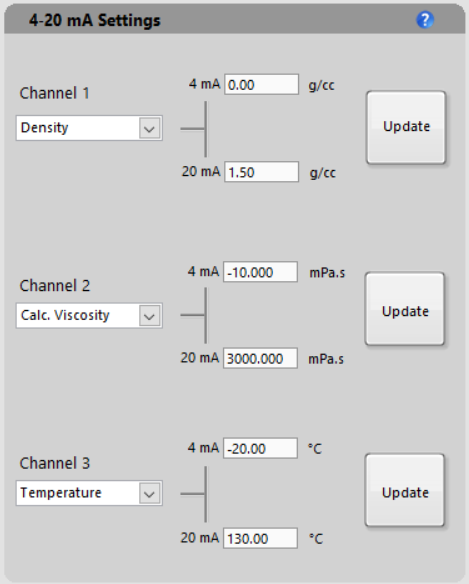Figure 10. Exponential viscosity loaded into the analog signal from the SMET.

`5.3. Modbus TCP/IP register`

Measurement can always be accessed at the register address that is displayed.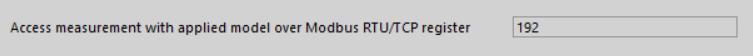The “Calculation History” button allows you to open a file where the last uploaded models and coefficients for these models are stored. This is for user reference.

# `6. Accesing compensated viscosity over RCP`

Users can access the mathematical calculation models after assigning an analog channel, reading a Modbus register, understanding the data stream, or setting the calculated values for exclusive readings with the RCP.

Below find the steps to display the temperature compensated viscosity on the “Measurement” Tab from the RCP.

`6.1. Go to the "Settings" tab and on the "Graph Settings" section open the Viscosity menu and select "Calc. Visc"`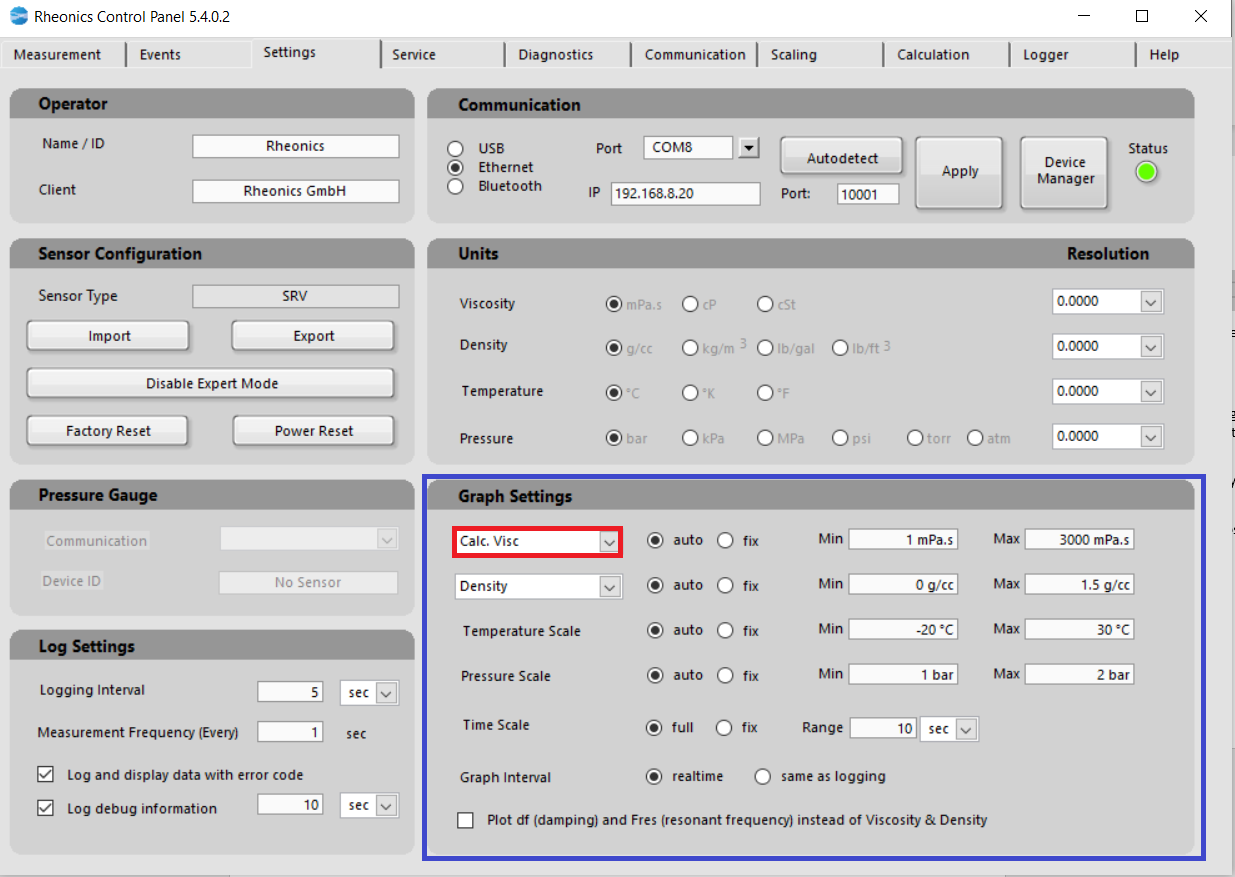Figure 11. RCP setup for special calculation models.

`6.2. With this selection, the "Measurement" tab is updated with the Calculated Viscosity instead of showing the standard parameter of viscosity`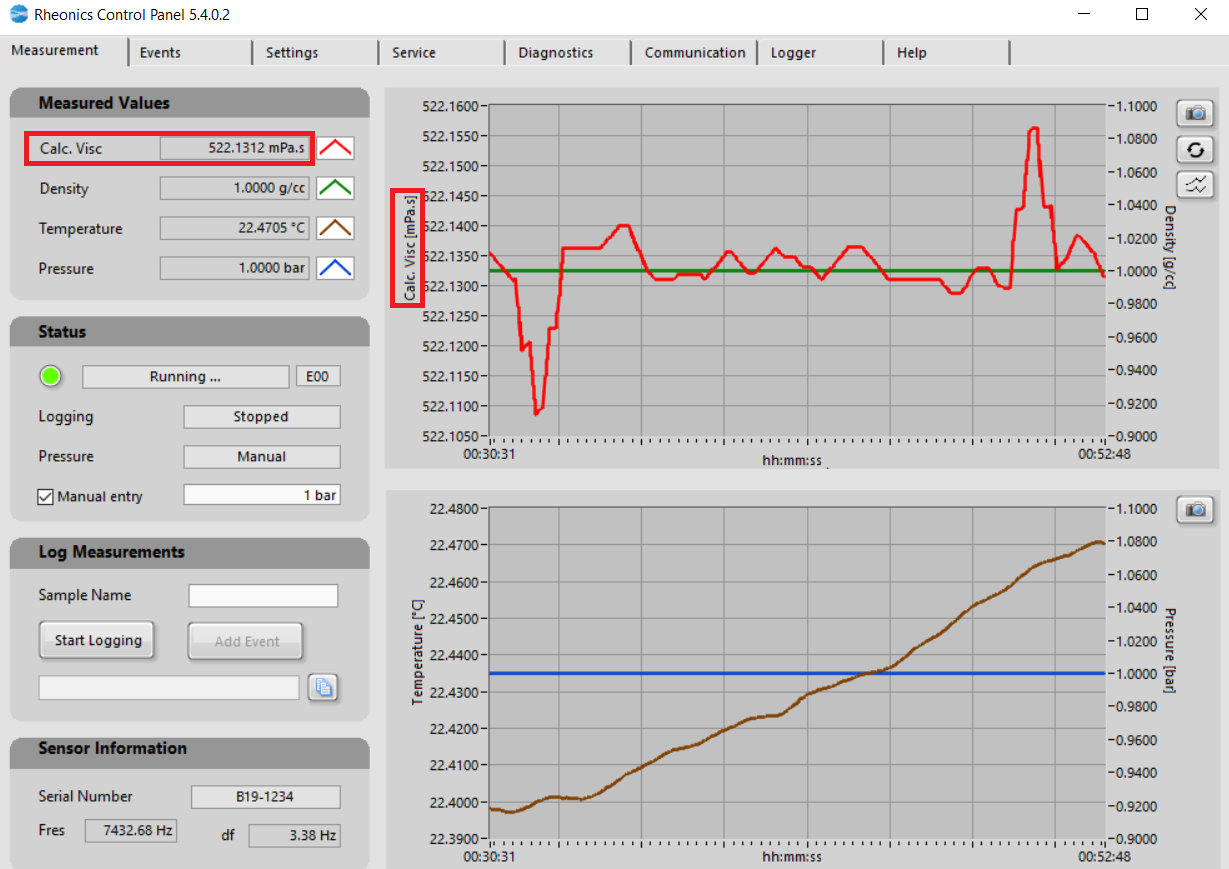Figure 12. Special calculation model loaded into “Measurement” tab.

# `7. Accessing line and compensated viscosity over digital channels.`

Rheonics offers a variety of communication protocols like Ethernet, Profinet, and Modbus, that can be used for further integration with other field devices.

`7.1. Modbus Register`

Measurement can always be accessed at the register address that is displayed. For compensated viscosity, this can be found at input register 192.https://support.rheonics.com/support/solutions/articles/81000397127-using-rheonics-file-for-settings-in-modbus`7.2. Profinet and Ethernet/IP address`

Parameter 19 stores the calculation model for viscosity, only one model for each variable can be loaded at a time.

 https://support.rheonics.com/support/solutions/articles/81000393235-parameter-list-access-for-field-devices# `8. Accessing both raw and compensated values through onboard logger`

Rheonics SMET (Electronics) comes with the ability to store measurement data on an SD-CARD. If the unit was ordered with SD-Card, then an industrial-grade SD-CARD card is already pre-inserted in the electronics.

Measurement and diagnostics data is stored on the card giving access to the last 1 year of data. The onboard data logger can be configured to store data for even longer periods of time and at an adjustable duty cycle.

Data can be saved by following the next steps:

1. Onboard logger can be accessed from the “Logger” tab, select the last date for the most recent logging and click the download button.
`8.1. Onboard logger can be accessed from the ""Logger" tab, select the last data for the most recent logging and click the download button.`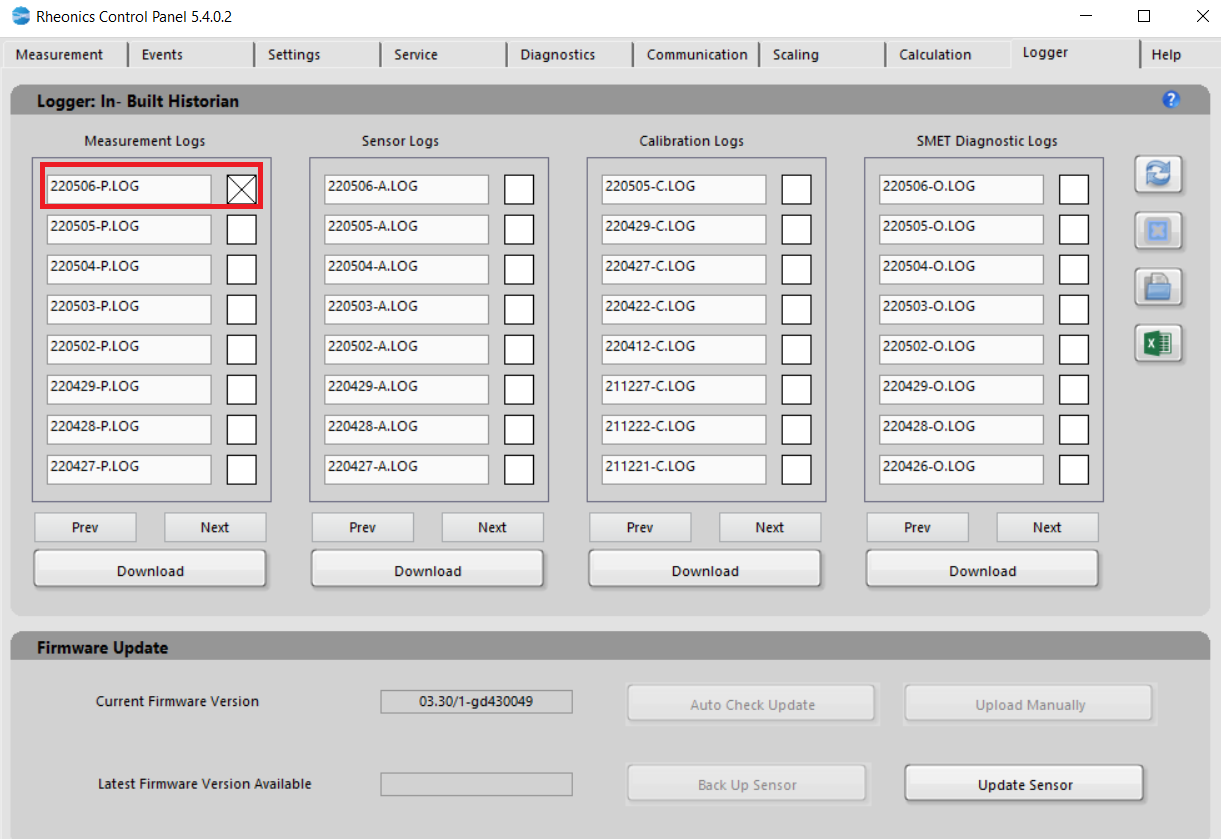Figure 13. Onboard Historian

1. Click on the “Excel” button, so the save data is directly loaded into an excel file.
`8.2. Click on the "Excel" button, so the save data directly loaded into the excel file.`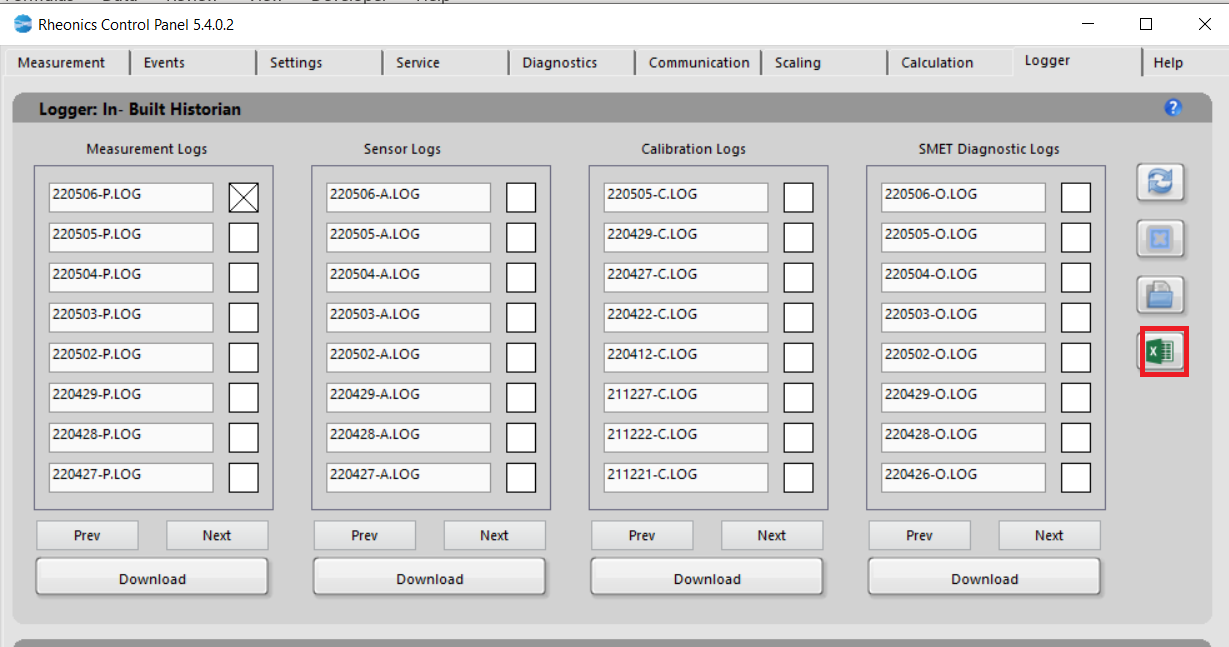Figure 14. Exporting data with the “Excel” button.

`8.3. Excel file is going to display all relevant data from each parameter provided.`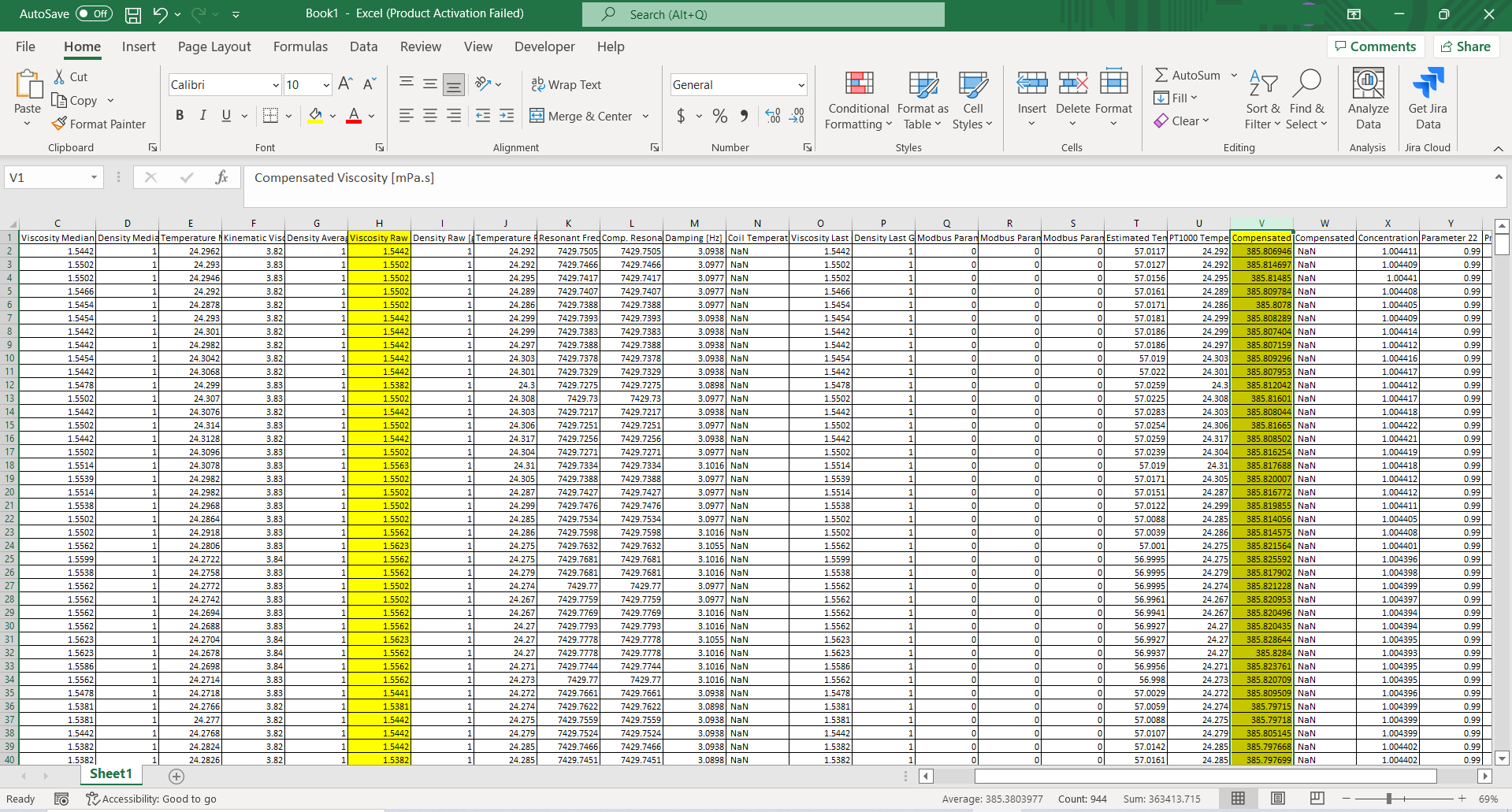Figure 15. How the data looks opened in excel.

Raw values correspond to parameter 5 Viscosity raw data and Calculated viscosity will correspond to parameter 19.

More detailed information about the onboard historian can be found at:

 https://support.rheonics.com/support/solutions/articles/81000383738-on-board-logger-historian-